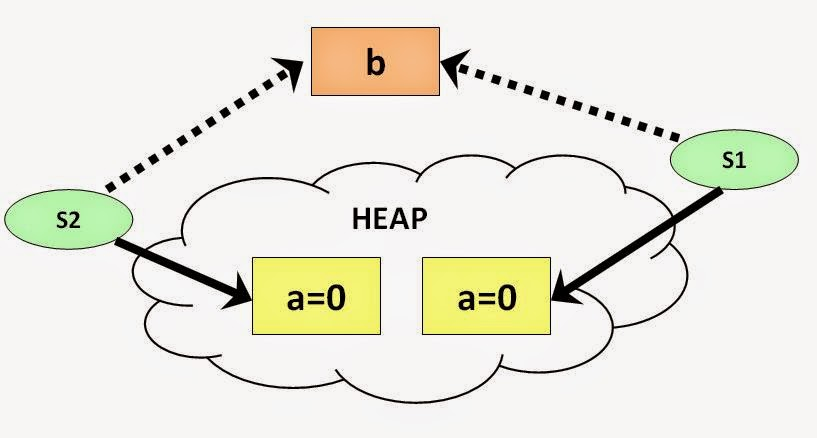Points To Remember
• Static variable are the variables at the class level.
• Static variables are not attached to the objects.
• Static variable cannot be declared inside any method of a class.
• Static variables are assigned the memory only once during the execution of the class.
• Static variables can be called directly from static context or by class reference.
Definition
Static variables are the class level variable that are allocated the memory only once and they cannot be declared inside any method of a class or inside a static block or static method but can be declared only  outside all methods and inside a class. A static variable is called by using the reference of the class and not the object.
ExampleSo lets assume an easy example where we have a class Test, with two variables, "a" non-static variable and the other "b" a static variable. Then variable "a" will be specific to the objects of the class Test and variable "b" will be common for all the objects of the class Test.

As shown in the image both object s1 and s2 have their own copy of variable "a", and both s1 and s2 have same copy of the variable "b".

`public class Test{  String a ;  static String b ;  public static void main(String args[]){  Test obj1 = new Test();  Test obj2 = new Test();  obj1.a = "Class A instance variable";  obj2.a = "Class B instance variable";  obj1.b = "Class A static variable";  obj2.b = "Class B static variable";  System.out.println(obj1.a);  System.out.println(obj2.a);  System.out.println(obj1.b);  System.out.println(obj2.b);  }}`
`Class A instance variableClass B instance variableClass B static variableClass B static variable`

So lets understand the output and match it with the statements we made above. An instance variable or non static variable is attached to an object (is at object level). This means that both objects obj1 and obj2 have their own variable "a". Thus obj1.a and obj2.a will give the values we assigned them. But when we assign static variable "b" value by obj1.b we assign value to the variable that is at class level. When we reassign it a value by obj2.b , the value of "b" is that was assigned by obj1.b is replaced by value of obj2.b. This is because static variable are at class level and only one copy of a static variable is created. Thus obj1.b and obj2.b both will point to the same variable.